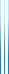# PublicationsPapers

## Comparison and equality queries achieve optimal redundancy

Yuval Dagan, Yuval Filmus and Shay Moran
Manuscript

Suppose that an element $x$ is sampled according to a known distribution $\mu$. Gilbert and Moore showed how to find $x$ using $H(\mu)+2$ comparison queries (in expectation).

In contrast, the classical Shannon–Fano code can recover $x$ using only $H(\mu)+1$ queries, but these queries can be arbitrary. Can we recover this result using a more restricted set of queries?

In this paper we show that by allowing equality queries, we can recover the performance of the Shannon–Fano code. We also determine the optimal number of queries needed to guarantee that $x$ can be recovered using $H(\mu)+r$ queries, for arbitrary $r\geq 1$. (Smaller $r$ cannot be achieved in general.)

This manuscript forms the first part of our more extensive STOC paper.